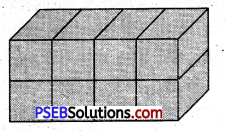# PSEB 5th Class Maths MCQ Chapter 9 Volume

Punjab State Board PSEB 5th Class Maths Book Solutions Chapter 9 Volume MCQ Questions and Answers.

## PSEB 5th Class Maths Chapter 9 Volume MCQ Questions

Multiple Choice Questions

Tick (✓) the correct answer :

Question 1.
Volume of cube with side 9 cm is :
(a) 81 cubic cm
(b) 90 cubic cm
(c) 729 cubic cm
(d) 8 cubic cm.
(c) 729 cubic cmQuestion 2.
Find volume of cuboid with length 6 cm, breadth 4 cm and height 2 cm :
(a) 24 cubic cm
(b) 28 cubic cm
(c) 64 cubic cm
(d) 48 cubic cm.
(d) 48 cubic cm.

Question 3.
Which is not the standard unit of volume?
(a) cubic cm
(b) sq. m
(c) cubic mm
(d) cubic metre.
(b) sq. m

Question 4.
A cuboid with all sides equal is called ……………
(a) square
(b) cube
(c) cuboid
(d) rectangle.
(b) cubeQuestion 5.
Count the number of cubes and find the volume of cuboid.Solution:
Volume of cuboid = 8 cubic cm.

Question 6.
A trolly is full of bricks. Length of the trolly is 400 cm, breadth is 200 cm and height is 100 cm. Length, breadth and height of a brick are 20 cm, 10 cm and 6 cm respectively. How many bricks are there in the trolly?
Solution:
Length of trolly, L = 400 cm
Breadth of trolly B = 200 cm
Height of trolly, H = 100 cm
Volume of trolly = L × B × H
= 400 cm × 200 cm × 100 cm.
Length of a brick, l = 20 cm
Breadth of a brick; b = 10 cm
Height of a brick, h = 6 cm
Volume of a brick = 20 cm × 10 cm × 6 cm
Number of bricks in the trolly
= $$\frac{\text { Volume of trolly }}{\text { Volume of each brick }}$$
= $$\frac{400 \times 200 \times 100}{20 \times 10 \times 6}$$
= $$\frac{20 \times 20 \times 50}{3}$$ = $$\frac{20000}{3}$$
= 6666 (Approx)